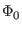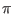Next: .noise Line Up: Analysis Specification Previous: .dc Line   Contents   Index

## .disto Line

The distortion analysis portion of WRspice computes steady-state harmonic and intermodulation products for small input signal magnitudes. If signals of a single frequency are specified as the input to the circuit, the complex values of the second and third harmonics are determined at every point in the circuit. If there are signals of two frequencies input to the circuit, the analysis finds the complex values of the circuit variables at the sum and difference of the input frequencies, and at the difference of the smaller frequency from the second harmonic of the larger frequency.

Distortion analysis is supported in WRspice only through residual incorporation from code imported from Berkeley SPICE3. This code is particularly complex, poorly documented, and ugly. Distortion analysis has not been tested, and may not work.

Distortion analysis is included for the following nonlinear devices: diodes, bipolar transistors, JFETs, MOSFETs (levels 1, 2, 3 and BSIM1) and MESFETS. All linear devices are automatically supported by distortion analysis. If there are switches present in the circuit, the analysis will continue to be accurate provided the switches do not change state under the small excitations used for distortion calculations.

General Form:
.disto dec|oct|lin np fstart fstop [f2overf1] [dc dc_args]
Examples:
.disto dec 10 1khz 100mhz
.disto dec 10 1khz 100mhz 0.9

A multi-dimensional Volterra series analysis is performed using a multi-dimensional Taylor series to represent the nonlinearities at the operating point. Terms of up to third order are used in the series expansions.

If the optional parameter f2overf1 is not specified, a harmonic analysis is performed -- i.e., distortion is analyzed in the circuit using only a single input frequency f1, which is swept as specified by arguments of the .disto line exactly as in an .ac line. Inputs at this frequency may be present at more than one input source, and their magnitudes and phases are specified by the arguments of the distof1 keyword in the input file lines for the input sources. The arguments of the distof2 keyword are not relevant in this case. The analysis produces information about the ac values of all node voltages and branch currents at the harmonic frequencies 2f1 and 3f1 , vs. the input frequency f1 as it is swept. A value of 1 (as a complex distortion output) signifies cos(2(2f1)t) at 2f1 and cos(2(3f1)t) at 3f1 , using the convention that 1 at the input fundamental frequency is equivalent to cos(2f1t) .

The distortion component desired (2f1 or 3f1 ) can be selected using commands in WRspice, and then printed or plotted. Normally, one is interested primarily in the magnitude of the harmonic components, so the magnitude of the ac distortion value is considered. It should be noted that these are the ac values of the actual harmonic components, and are not equal to HD2 and HD3. To obtain HD2 and HD3, one must divide by the corresponding ac values at f1, obtained from an .ac line. This division can be done using WRspice commands.

If the optional f2overf1 parameter is specified, it should be a real number between (and not equal to) 0.0 and 1.0; in this case, a spectral analysis is performed. The circuit is considered with sinusoidal inputs at two different frequencies f1 and f2. Frequency f1 is swept according to the .disto line options exactly as for the .ac card. Frequency f2 is kept fixed at a single frequency as f1 sweeps -- the value at which it is kept fixed is equal to f2overf1*fstart. Each voltage and current source in the circuit may potentially have two (superimposed) sinusoidal inputs for distortion, at the frequencies f1 and f2. The magnitude and phase of the f1 component are specified by the arguments of the distof1 keyword in the source's input line, as described in 2.15; the magnitude and phase of the f2 component are specified by the arguments of the distof2 keyword. The analysis produces plots of all node voltages/branch currents at the intermodulation product frequencies f1 + f2 , f1 - f2 , and (2f1) - f2 , vs the swept frequency f1. The IM product of interest may be selected using the setplot command, and displayed with the print and plot commands. As in the harmonic analysis case, the results are the actual ac voltages and currents at the intermodulation frequencies, and need to be normalized with respect to .ac values to obtain the IM parameters.

If the distof1 or distof2 keywords are missing from the description of a voltage or current source, then that source is assumed to have no input at the corresponding frequency. The default values of the magnitude and phase are 1.0 and 0.0 respectively. The phase should be specified in degrees.

It should be noted that the number f2overf1 should ideally be an irrational number, and that since this is not possible in practice, efforts should be made to keep the denominator in its fractional representation as large as possible, certainly above 3, for accurate results. If f2overf1 is represented as a fraction A/B , where A and B are integers with no common factors, B should be as large as possible. Note that A < B because f2overf1 is constrained to be < 1). To illustrate why, consider the cases where f2overf1 is 49/100 and 1/2. In a spectral analysis, the outputs produced are at f1 + f2 , f1 - f2 and 2f1 - f2 . In the latter case, f1 - f2 = f2 , so the result at the f1 - f2 component is erroneous because there is the strong fundamental f2 component at the same frequency. Also, f1 + f2 = 2f1 - f2 in the latter case, and each result is erroneous individually. This problem is not seen in the case where f2overf1 = 49/100 , because f1 - f2 = 51/100f1 which is not equal to 49/100f1 = f2 . In this case, there will be two very closely spaced frequency components at f2 and f1 - f2 . One of the advantages of the Volterra series technique is that it computes distortions at mix frequencies expressed symbolically (i.e. n . f1m . f2), therefore one is able to obtain the strengths of distortion components accurately even if the separation between them is very small, as opposed to transient analysis for example. The disadvantage is of course that if two of the mix frequencies coincide, the results are not merged together and presented, though this could presumably be done as a postprocessing step. Currently, the interested user should keep track of the mix frequencies and add the distortions at coinciding mix frequencies together should it be necessary.

The optional dc sweep is a dc analysis specification which will cause the distortion analysis to be performed at each point of the dc sweep. The small-signal parameters are reevaluated at every sweep point, and the output vectors will be multidimensional.

In interactive mode, the disto command, which takes the same arguments as the .disto line, can be used to initiate distortion analysis.

Distortion analysis is not available if Josephson junctions are included in the circuit.Next: .noise Line Up: Analysis Specification Previous: .dc Line   Contents   Index
Stephen R. Whiteley 2020-07-23